Problem

# If a force of P = 200 N is applied to the handle of the bell crank, determine the maximum...

If a force of P = 200 N is applied to the handle of the bell crank, determine the maximum torque M that can be resisted so that the flywheel does not rotate clockwise. The coefficient of static friction between the brake band and the rim of the wheel is μs = 0.3.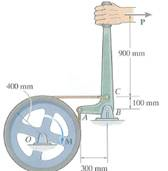#### Step-by-Step Solution

Solution 1

Given:-

Force,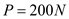Coefficient of static friction between brake bad and rim,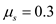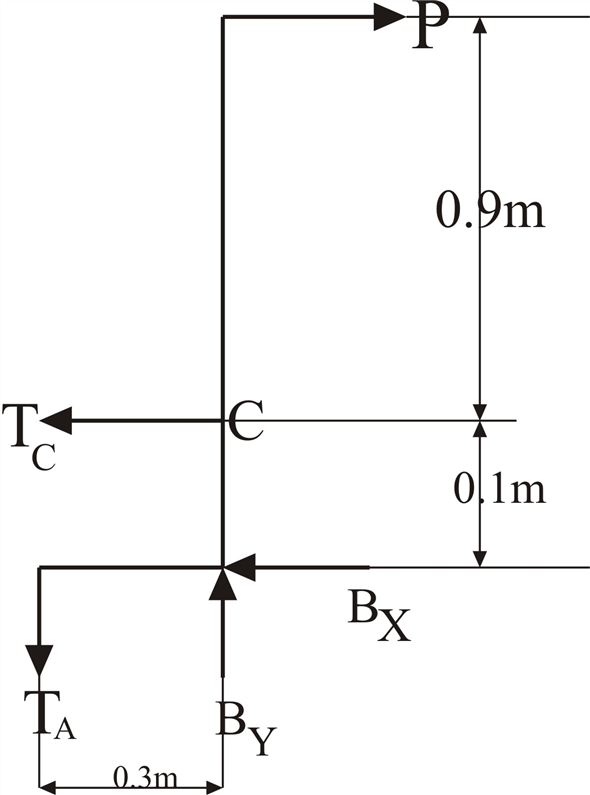(a)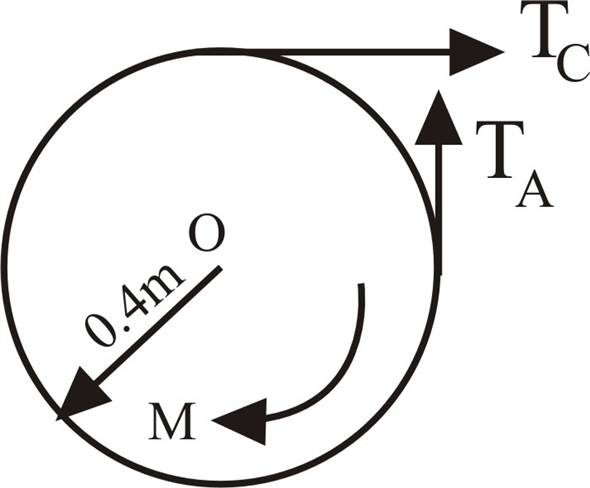(b)

From figure (A):

Take moment about ‘B’: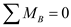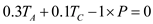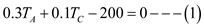From Figure (B):

Take moment about ‘O’: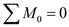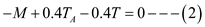Angle of contact,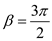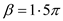We know that,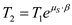Here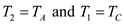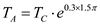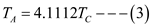From (1) & (3)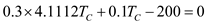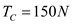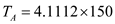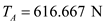From (2)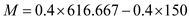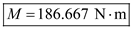Add your Solution
Textbook Solutions and Answers Search
Solutions For Problems in Chapter 8